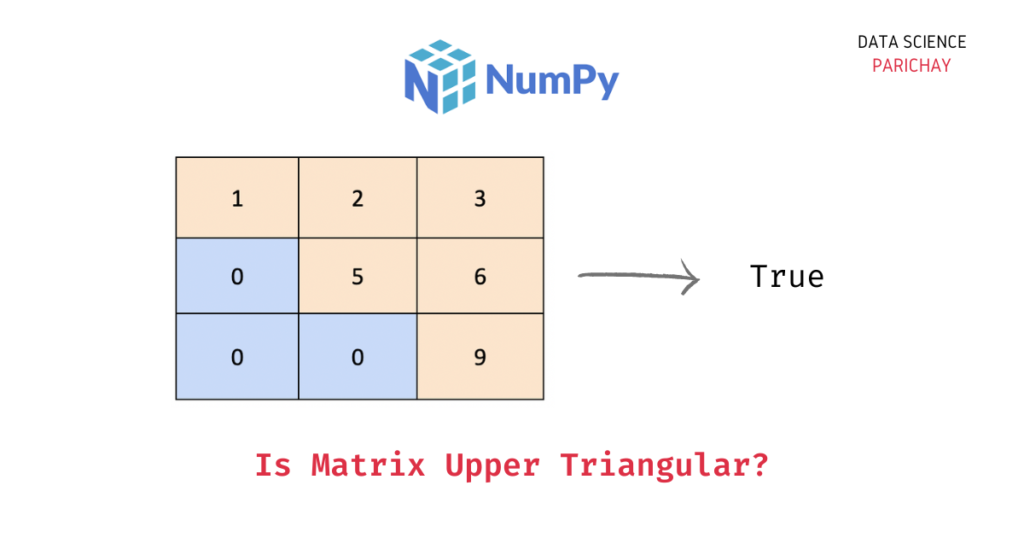# Numpy – Check if Matrix is an Upper Triangular Matrix

In this tutorial, we will look at how to check if a numpy matrix (a 2d numpy array) is an upper triangular matrix or not with the help of some examples.

### What is an upper triangular matrix?

A matrix is considered an upper triangular matrix if all the elements below the main diagonal are zero. The following image shows an upper triangular matrix.

Notice that all the elements below the main diagonal are zero.

## How to check if a matrix is upper triangular in Numpy?

To check if a matrix is upper triangular or not, compare the original matrix with the upper triangular matrix generated from the matrix, if both the matrices are the same, we can say that the original matrix is an upper triangular matrix.

Use the following steps –

1. Use the `numpy.triu()` function to generate the upper triangular matrix resulting from the original matrix.
2. Compare if the above-generated matrix is the same as the original matrix. You can use the `numpy.array_equal()` function to compare two arrays for equality. If the matrices are equal, the original matrix is upper triangular.

The following is the syntax –

```import numpy as np

# check if the matrix ar is upper triangular
np.array_equal(ar, np.triu(ar))```

Let’s now look at some examples of using the above syntax –

### Example 1 – Check if a square matrix is upper triangular

Let’s create an upper triangular square matrix and check if the above method identifies it correctly or not.

📚 Data Science Programs By Skill Level

Introductory

Intermediate ⭐⭐⭐

🔎 Find Data Science Programs 👨‍💻 111,889 already enrolled

Disclaimer: Data Science Parichay is reader supported. When you purchase a course through a link on this site, we may earn a small commission at no additional cost to you. Earned commissions help support this website and its team of writers.

```import numpy as np

# create an upper triangular matrix
ar = np.array([
[1, 2, 3],
[0, 5, 6],
[0, 0, 9]
])

# check if the above matrix is upper triangular
print(np.array_equal(ar, np.triu(ar)))```

Output:

`True`

Let’s look at an example where the matrix is not an upper triangular matrix.

```import numpy as np

# create a matrix
ar = np.array([
[1, 2, 3],
[4, 5, 6],
[7, 0, 9]
])

# check if the above matrix is upper triangular
print(np.array_equal(ar, np.triu(ar)))```

Output:

`False`

We get `False` as the output since in the above matrix there are non-zero elements below the main diagonal, that is, it is not an upper triangular matrix.

### Example 2 – Check if a non-square matrix is upper triangular

We can also use this method on non-square matrices (where the number of rows and columns is not equal). Let’s look at an example.

```import numpy as np

# create an upper triangular matrix
ar = np.array([
[1, 2, 3],
[0, 5, 6],
[0, 0, 9],
[0, 0, 0]
])

# check if the above matrix is upper triangular
print(np.array_equal(ar, np.triu(ar)))```

Output:

`True`

The matrix in the above example is a 4×3 matrix (4 rows and 3 columns). We get `True` as the output because it’s an upper triangular matrix (all the elements below the main diagonal 1-5-9 are zero).

Let’s look at another example.

```import numpy as np

# create an upper triangular matrix
ar = np.array([
[1, 2, 3],
[0, 5, 6],
])

# check if the above matrix is upper triangular
print(np.array_equal(ar, np.triu(ar)))```

Output:

`True`

Here, we create a 2×3 matrix. We get `True` as the output because it’s an upper triangular matrix (all the elements below the main diagonal, 1-5 are zero).

You might also be interested in –

•